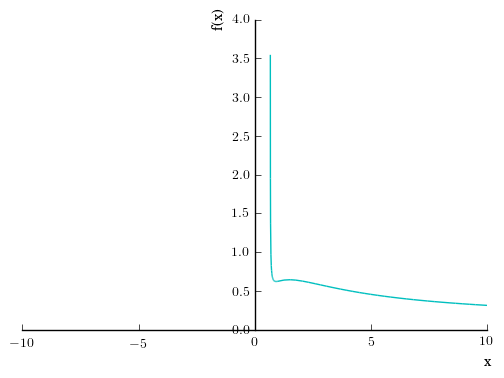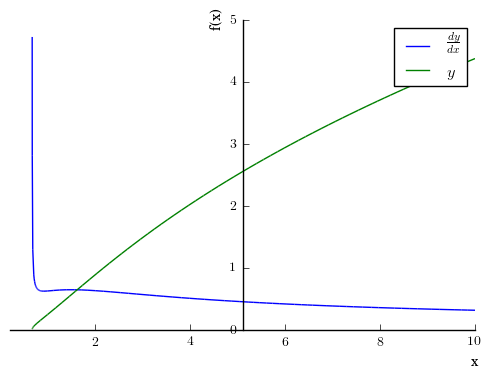This is a quick demo of Sympy, partly for you, but mostly for me. The actual notebook is available on my github iPythonExamples repository.

# import symbolic capability to Python
from sympy import *

# print things all pretty
from sympy.abc import *
init_printing()


Consider solving the problem

$$y = \frac{x^2\sqrt{3x-2}}{(x+1)^2}$$, find $$\frac{dy}{dx}$$

# Need to define variables as symbolic for sympy to use them.
x, y= symbols("x, y", real = True)

# Define y
y = x**2*sqrt(3*x-2)/(x+1)**2
y # This causes it to output formatted nicely. The print command formats it as computer code

\begin{equation*} \frac{x^{2} \sqrt{3 x - 2}}{\left(x + 1\right)^{2}} \end{equation*}
# Taking the natural log of this:
a = ln(x**2*sqrt(3*x-2)/(x+1)**2)
a # Keep in mind that the left hand side is ln(y)

\begin{equation*} \log{\left (\frac{x^{2} \sqrt{3 x - 2}}{\left(x + 1\right)^{2}} \right )} \end{equation*}
# Taking its derivative with respect to x
# So, we took the log of the right side, then the derivative
diff(a,x)

\begin{equation*} \frac{\left(x + 1\right)^{2}}{x^{2} \sqrt{3 x - 2}} \left(\frac{3 x^{2}}{2 \left(x + 1\right)^{2} \sqrt{3 x - 2}} - \frac{2 x^{2} \sqrt{3 x - 2}}{\left(x + 1\right)^{3}} + \frac{2 x \sqrt{3 x - 2}}{\left(x + 1\right)^{2}}\right) \end{equation*}
# That is kind of messy. Let the computer simplify it
simplify(diff(a,x))

\begin{equation*} \frac{3 x^{2} + 15 x - 8}{2 x \left(3 x^{2} + x - 2\right)} \end{equation*}
# We could have done this all at once
simplify(diff(ln(y),x))

\begin{equation*} \frac{3 x^{2} + 15 x - 8}{2 x \left(3 x^{2} + x - 2\right)} \end{equation*}

Now, the left hand side processed the same way is

\begin{equation*} y \end{equation*}

take the natural log

\begin{equation*} \ln(y) \end{equation*}

Take the derivative

\begin{equation*} \frac{1}{y}dy \end{equation*}

So, our entire processed equation is

\begin{equation*} \frac{1}{y}dy = \frac{3 x^{2} + 15 x - 8}{2 x \left(3 x^{2} + x - 2\right)} \end{equation*}

so all we have to do is multiply the processed right side by $$y$$ and we have our answer and our derivative is

simplify(diff(ln(y),x)*y)

\begin{equation*} \frac{x \left(3 x^{2} + 15 x - 8\right)}{2 \sqrt{3 x - 2} \left(x^{3} + 3 x^{2} + 3 x + 1\right)} \end{equation*}

Of course, Sympy is capable enough that we can just take the derivative directly

simplify(diff(y,x))

\begin{equation*} \frac{x \left(3 x^{2} + 15 x - 8\right)}{2 \sqrt{3 x - 2} \left(x^{3} + 3 x^{2} + 3 x + 1\right)} \end{equation*}

but that was way too easy.

We could compare them, just to be sure they are the same

simplify(diff(y,x))==simplify(diff(ln(y),x)*y)

True


Or plot this monstrosity

plot((diff(y,x),(x,0.2,10)), (y, (x,0.5,10)))# To change colors
# show = False delays the plot until we can set all of the parameters
# legend turns on the legend and uses the labels we have later.
p = plot((diff(y,x),(x,0.2,10)), (y, (x,0.5,10)), show = False, legend = True)
p.line_color = 'blue'
p.label = '$\\frac{dy}{dx}$'
p.line_color = 'green'
p.label = '$y$'
p.show()What if we wanted to make a substitution?

y.subs(x,alpha)

\begin{equation*} \frac{\alpha^{2} \sqrt{3 \alpha - 2}}{\left(\alpha + 1\right)^{2}} \end{equation*}